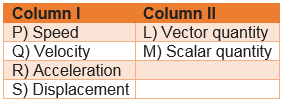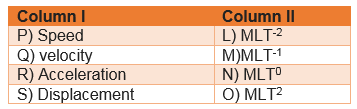Q.1
##### A particles moves with a constant velocity STATEMENT -1: Acceleration is zero for the particle STATEMENT -2: Average velocity is equal to instantaneous velocity STATEMENT -3: Average speed is equal to magnitude of instantaneous velocity STATEMENT -4: Position-time graph for the particle is a straight line which one of the following is correct?
• All the statement are correct
• Statement 1,2 and 4 are correct
• Statement 1,2 and 3 are correct
• Statement 2,3 and 4 are correct
Q.2
##### Match the column• P->M,Q->L,R->L,S->L
• P->L,Q->M,R->L,S->L
• P->M,Q->M,R->L,S->L
• P->M,Q->L,R->M,S->L
Q.3
• True
• False
Q.4
##### Two balls of different masses are dropped from the same point at same time.Which is of the following is false?
• Both the balls will reach the ground in same time
• Heavier ball will hit the ground first
• Both the balls will strike the ground with the same speed
• None of these
Q.5
##### Which of following statement is Incorrect?
• An object with a positive velocity will be represented on a position-time graph by a line with a positive slope
• An object with a negative velocity will be represented on a position-time graph by a line with a negative slope
• An object with zero velocity will be represented on a position-time graph by a line with zero slope
• None of these
Q.6
• True
• False
Q.7
• A
• B
• C
• D
Q.8
• True
• False
Q.9
##### Two statement are made STATEMENT -A: Displacement of the particle may be zero even if the distance covered by it is not zero STATEMENT -B: Displacement is the shortest distance between the initial and final position
• Both the statement are correct
• A is correct but B is incorrect
• A is incorrect but B is correct
• Both are incorrect
Q.10
##### Which of following statement is correct?
• The magnitude of the instantaneous velocity is equal to the instantaneous speed
• The magnitude of the average velocity is equal to the average speed
• Average acceleration is equal to change is speed per unit time
• none of these
Q.11
##### Mark the wrong statement
• Magnitude of displacement is always equal to distance traveled
• Area under velocity versus time graph with proper algebraic sign gives the displacement
• Speed can not be negative
• If velocity is constant,speed will be constant
Q.12
##### Match the column• P->M,Q->L,R->L,S->L
• P->M,Q->M,R->L,S->N
• P->M,Q->M,R->L,S->O
• P->M,Q->N,R->M,S->O
Q.13
• True
• False
Q.14
##### Which of the following option is correct?
• Mean Velocity=(vf + vi )/2
• Average acceleration =(vf - vi )/(t2 -t1)
• Displacement in a motion in a straight line with uniform acceleration is given by s=u + (1/2)at2
• None of these
Q.15
##### Two statement are made STATEMENT A: Average speed is not equal to the magnitude of average velocity in general STATEMENT B: Average velocity is a scalar while average speed is a vector quantity
• Both the statement are correct
• Statement A is correct only
• Statement B is correct only
• Both the statement A and B both are wrong
Q.16
##### A body is thrown upwards with certain velocity.Which of the following is Incorrect
• The body will experience retardation
• The body velocity will become zero at the peak point
• The time taken to reach the peak point is u/g
• None of these
Q.17
##### Instantaneous acceleration is defined as
• $\frac {dx}{dt}$
• $\frac {d^2x}{dt^2}$
• $\frac { \Delta x}{ \Delta t}$
• None of these
Q.18
• True
• False
Q.19
##### Four statement are made for acceleration STATEMENT -1: Acceleration is a vector quantity STATEMENT -2: The slope of the velocity time graph gives the acceleration STATEMENT -3: Its direction is same as the change in velocity STATEMENT -4: $a=v \frac {dv}{dx}$ which one of the following is correct
• All the statement are correct
• Statement 1,2 and 4 are correct
• Statement 1,2 and 3 are correct
• Statement 2,3 and 4 are correct
Q.20
##### At a metro station, a girl walks up a stationary escalator in time a​. If she remains stationary on the escalator, then the escalator take her up in time b​. The time taken by her to walk up on the moving escalator will be
• $\frac {a+b}{2}$
• a+b
• $\frac {ab}{a- b}$
• $\frac {ab}{a+ b}$